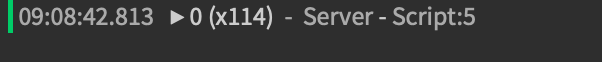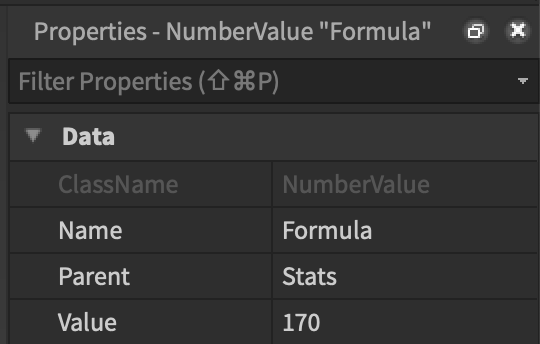# Value printing as 159, 166, and now 0 even though it is clearly 170

## Value printing as 159, 166, and now 0 even though it is clearly 170.

Hello, recently my friend has been adding some scripts to create a leveling system for his game “Don’t Fall!” However, he has run into a problem that neither he nor I can get past. Whenever we try to transport the “formula”, a value determined by players stats to determine how much experience a player needs to level up, the value prints differently. First, it printed 159, when “formula” was 170. After that, it started printing 166. Now, it’s printing 0, even though player.Stats.Formula.Value is clearly 170.
We have tried everything. Can someone explain what’s happening?

ImagesCan you send the script? If you are using events then I think I know what the problem is

Are you sure you’re not changing the value locally? Also there isn’t much we can do without seeing the code.

1 Like

This is most likely a client → server replication issue, meaning the value isn’t being changed on the server because its being changed on the client

GUI:

``````local player = script.Parent.Parent.Parent.Parent
local formula = player.Stats.Formula.Value
while wait(0.5) do
script.Parent.Text = "EXP: ".. player.leaderstats.EXP.Value .. " / ".. formula
print(formula)
end
``````

Data:

``````local formula = Instance.new("NumberValue", s)
formula.Name = "Formula"
formula.Value = tonumber(math.floor(150 + (16.2 + (le.Value * le.Value))))

while wait(0.08) do
formula.Value = math.floor(150 + (16.2 + (le.Value * le.Value)))
le.Value = MainOperations.level(formula.Value, le.Value, e.Value)
e.Value = MainOperations.exp(formula.Value, le.Value, e.Value)
end
``````

Function/Main operations:

``````function MainOperations.en(player)
local GUI = game.ServerStorage.GUI
local e = GUI:Clone()
e.Parent = player.PlayerGui
local random1 = math.random(1,70)
e.ImageLabel.Position = UDim2.new(random1/100, 0, random1/100, 0)
wait(2)
e:Destroy()
end
function MainOperations.level(formula, level, exp)
if exp > formula then
level += 1
end
return level
end
function MainOperations.exp(formula, level, exp)
if exp > formula then
exp -= formula
end
return exp
end
return MainOperations
``````

GUI is printing the random numbers (159,166,0)
Data and main operations print 170 like it is supposed to.

Can you specify whether your code runs on the server or client?

You’re defining the initial value of the BaseValue “Formula”. You should only add .Value when you want to use the actual value of the object. Basically you’re storing the number 0 (which is what the original value of variable formula was) in the variable “formula”. So every time you reference, you’re simply referencing the same number (0) each time

Just add the .Value in the while loop

``````local player = script.Parent.Parent.Parent.Parent
local formula = player.Stats.Formula
while wait(0.5) do
script.Parent.Text = "EXP: ".. player.leaderstats.EXP.Value .. " / ".. formula.Value
print(formula)
end
``````
1 Like

All scripts are server scripts except the function is a module.

That worked! Thank you. Lua is so demanding lol.